maths > construction-high

Construction of Triangles With Secondary Information

what you'll learn...

overview

Triangles are defined by $3$$3$ independent parameters. Usually, the three independent parameters are chosen from $6$$6$ primary parameters -- the three sides and the three angles.

The sum of two sides, or perimeter of the triangle, etc. are secondary parameters.

If one or more of given parameters is of secondary type, then how to construct the specified triangle? A short overview of approaching such problems is provided in this lesson.

An overview of constructing triangles with angle-side-sum of two sides is provided. Sum of two sides is a secondary parameter.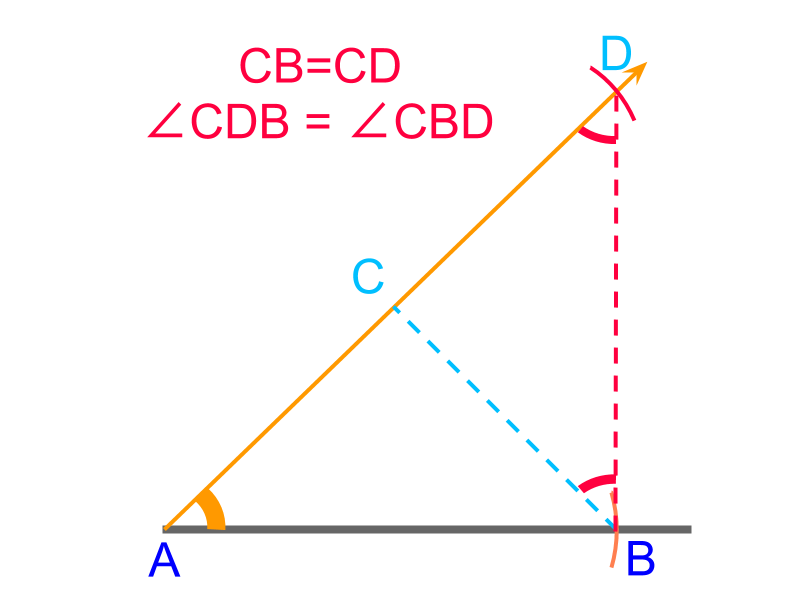An overview of constructing triangles with angle-side-difference between two sides is provided. Difference between two sides is a secondary parameter.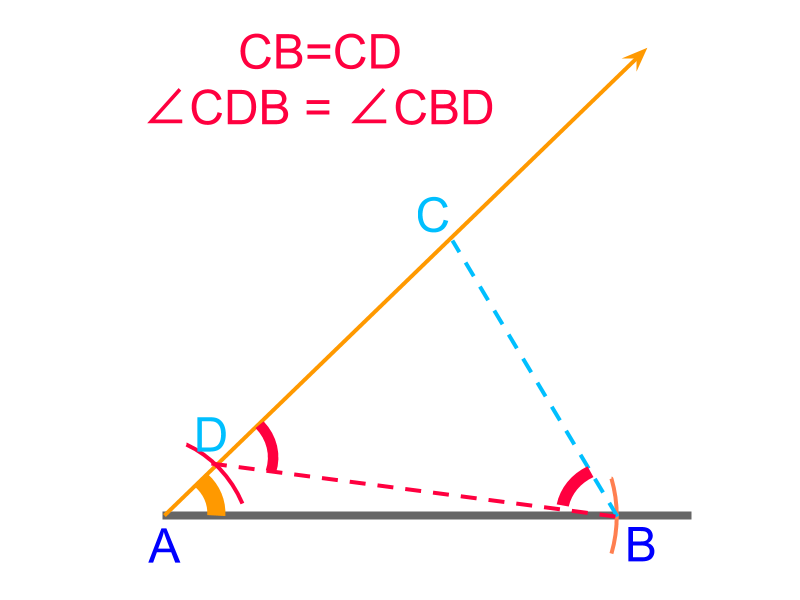An overview of constructing triangles with angle-side-negative difference between two sides is provided. Negative difference between two sides is a secondary parameter.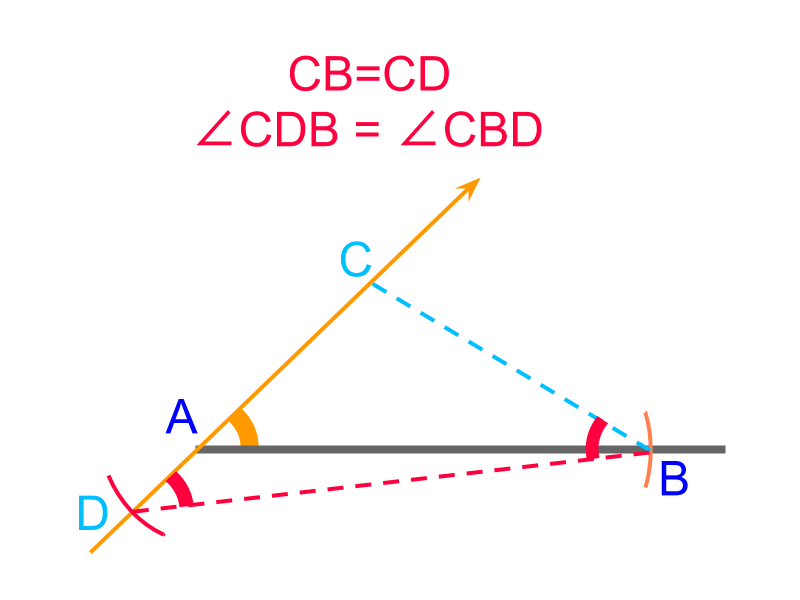An overview of constructing triangles with angle-angle-perimeter is provided. Perimeter is a secondary parameter.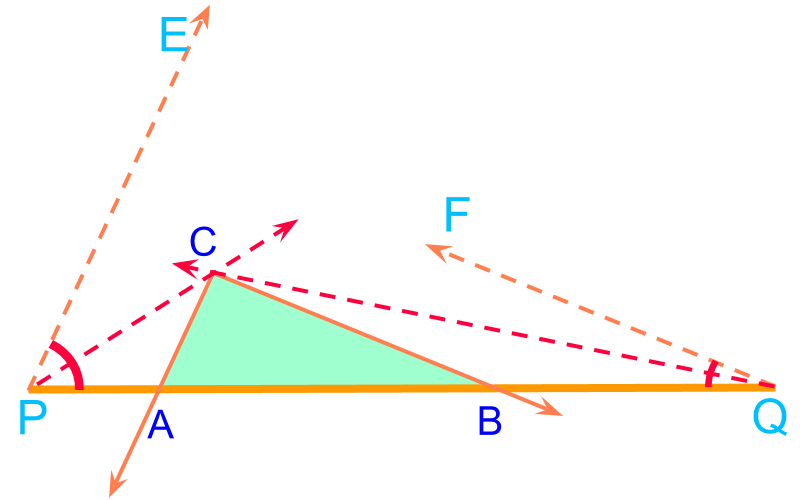recap

To construct any shape, the fundamental elements we use are

•  Construct a line passing through the two given points using a ruler or scale (collinear points)

•  Construct a ray at the given angle using a protractor (equiangular points)

•  Construct an arc at the given distance using a compass and a ruler or scale (equidistant points)

•  construct a parallel line using a set-square and a scale or another set-square. (points on a parallel)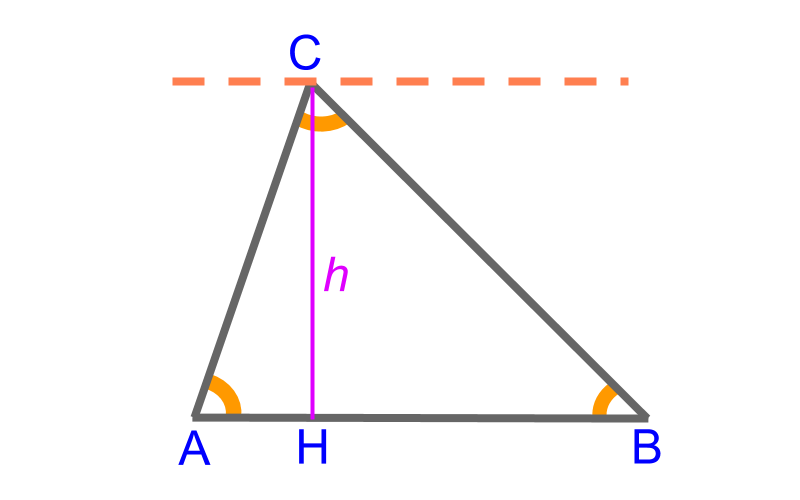Triangle $△ABC$$\triangle A B C$ has the following measurements as the parameters

•  side $\overline{AB}$$\overline{A B}$

•  side $\overline{BC}$$\overline{B C}$

•  side $\overline{CA}$$\overline{C A}$

•  angle $\angle A$$\angle A$

•  angle $\angle B$$\angle B$

•  angle $\angle C$$\angle C$

•  altitude or height of triangle $\overline{CH}=l$$\overline{C H} = l$

These are $7$$7$ parameters in total. Among these $7$$7$, any $3$$3$ independent parameters define a triangle. Other parameters can be derived from the given three parameters.

Note that the three angles are not truly independent as $\angle A+\angle B+\angle C={180}^{\circ }$$\angle A + \angle B + \angle C = {180}^{\circ}$ These parameters are basic parameters.

Earlier we studied about construction of triangles. To construct a triangle, one of the following set of parameters is provided.

•  side-side-side (sss)

•  side-angle-side (sas)

•  angle-side-angle (asa)

In these, each of the parameters directly provides one of $3$$3$ sides or $3$$3$ angles

derived parameters

Apart from the basic parameters, a derived parameter can be provided. Examples of "derived" parameters are
sum of two sides
difference between two sides
perimeter of the triangle

If the following are provided, how to construct the triangle?
side-angle-sum of two sides
side-angle-difference between two sides
perimeter-angle-angle

With trigonometry or with coordinate geometry, the given parameters can be converted to basic parameters. But, the objective of geometrical construction is to figure out the shape (the triangle) without any calculations.

In this lesson, construction of triangles with derived or secondary parameters is explained.

side-angle-sum of two sides

To construct a triangle, "side-angle-sum of other two sides" is provided. The given angle with two line segments of given side and sum of sides are directly constructed using the given parameters.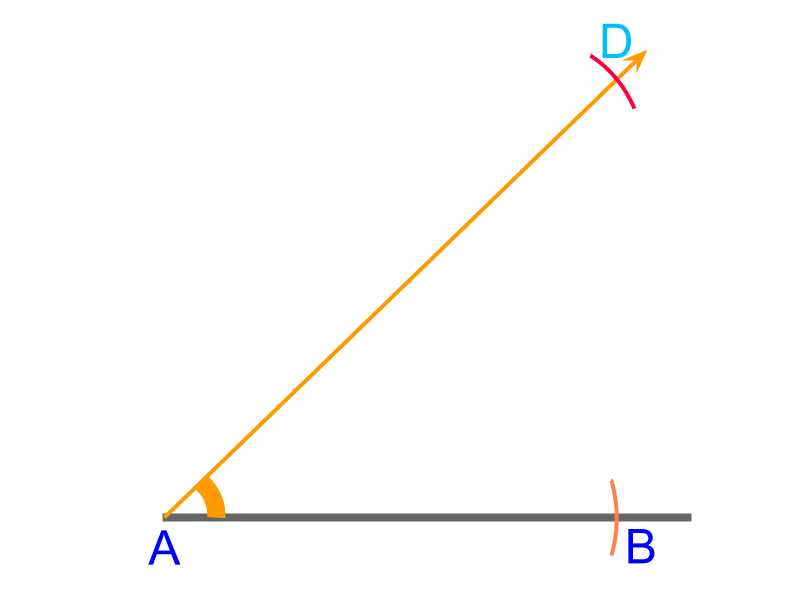To construct a triangle $△ABC$$\triangle A B C$ with given "side-angle-sum of other two sides" $\overline{AB}=4$$\overline{A B} = 4$cm, $\angle A={50}^{\circ }$$\angle A = {50}^{\circ}$, and $\overline{AC}+\overline{BC}=6$$\overline{A C} + \overline{B C} = 6$cm.

•  Construct a line and mark side $\overline{AB}$$\overline{A B}$ with a compass measuring $4$$4$cm

•  measure ${50}^{\circ }$${50}^{\circ}$ angle and draw a ray from point $A$$A$

•  measure $\overline{AC}+\overline{BC}$$\overline{A C} + \overline{B C}$ $=6$$= 6$cm in a compass and draw an arc cutting the ray at $D$$D$

This is directly constructed from the given parameters.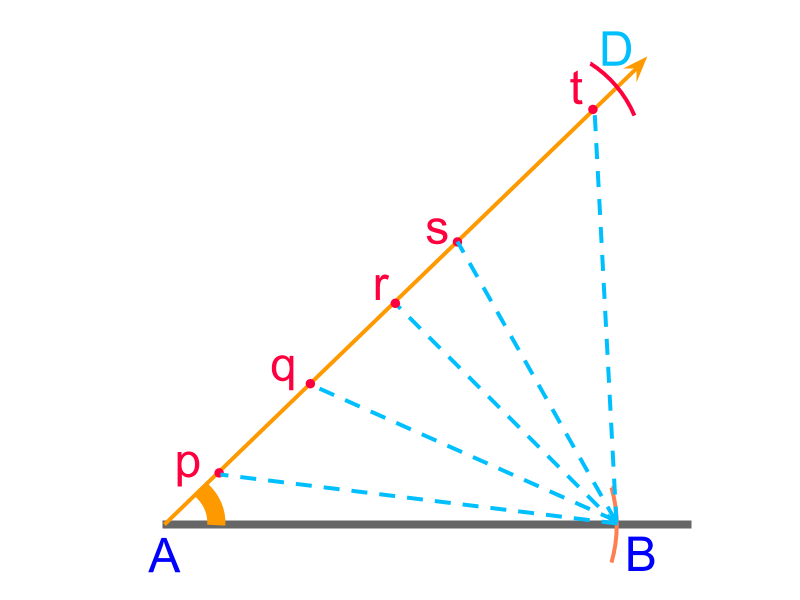With given "side-angle-sum of two sides" we constructed $\overline{AB}$$\overline{A B}$, and $\overline{AD}=\overline{AC}+\overline{CB}$$\overline{A D} = \overline{A C} + \overline{C B}$ at the given angle $\angle A$$\angle A$. The objective now is to locate the point $C$$C$ in the line $\overline{AD}$$\overline{A D}$.

Consider points $p$$p$, $q$$q$, $r$$r$, $s$$s$, and $t$$t$ on line $\overline{AD}$$\overline{A D}$. The following are observed.

•  $\overline{Ap}+\overline{pB}<\overline{AD}$$\overline{A p} + \overline{p B} < \overline{A D}$

•  $\overline{At}+\overline{tB}>\overline{AD}$$\overline{A t} + \overline{t B} > \overline{A D}$

•  It is noted that at a single position between the points $A$$A$ and $D$$D$, the point $C$$C$ is located such that $\overline{AC}+\overline{CB}=\overline{AD}$$\overline{A C} + \overline{C B} = \overline{A D}$.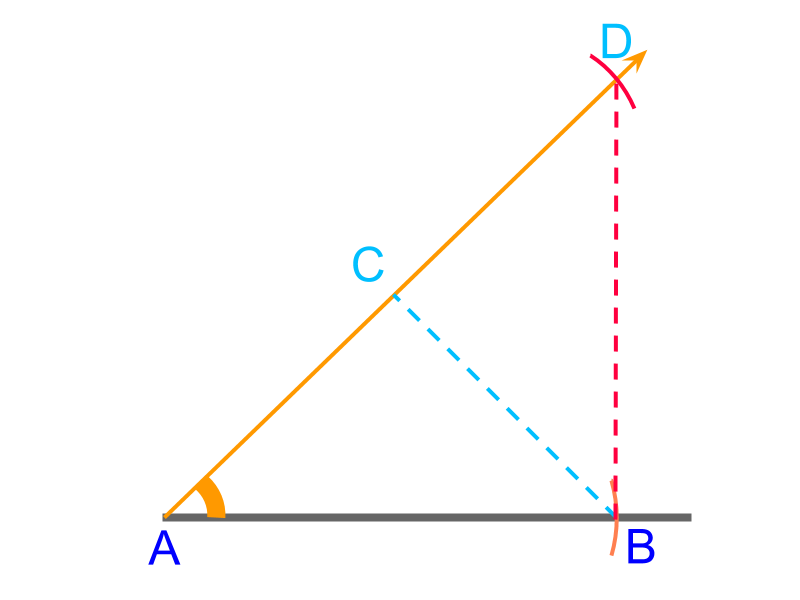The point $C$$C$ is visualized in $\overline{AD}$$\overline{A D}$ such that $\overline{AC}+\overline{CB}=\overline{AD}$$\overline{A C} + \overline{C B} = \overline{A D}$. The line segments $\overline{CD}$$\overline{C D}$ and $\overline{CB}$$\overline{C B}$ are equal, as the triangle $△BCD$$\triangle B C D$ is isoscelesIt is observed that $△BCD$$\triangle B C D$ is isosceles and only angle $\angle D$$\angle D$ and base $\overline{BD}$$\overline{B D}$ are available. The position of point $C$$C$ is not yet marked. It is shown in the figure to visualize. To help in identifying the point $C$$C$, one the following properties is used :

•  two angles of an isosceles triangles are equal.

•  a perpendicular bisector on the base of isosceles triangle passes through the third vertex.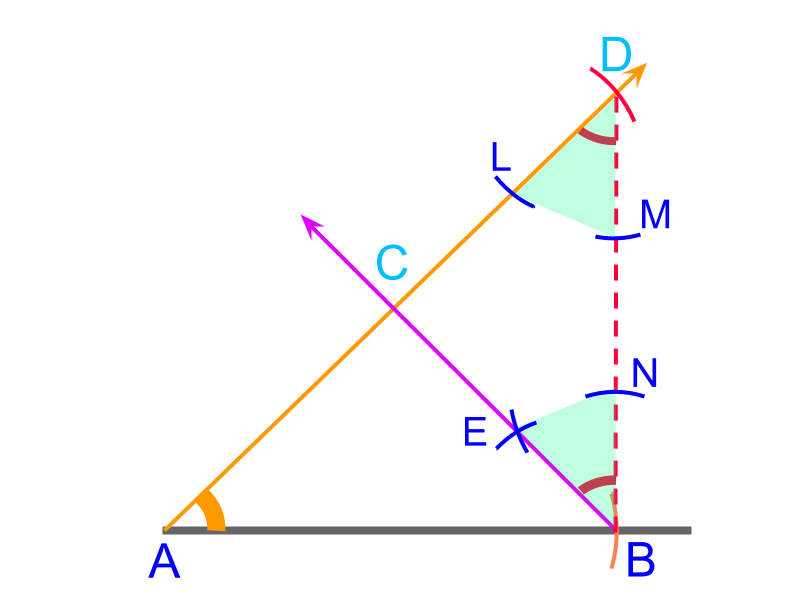Using the property that
two angles of an isosceles triangles are equal. $\angle CDB=\angle DBC$$\angle C D B = \angle D B C$
The angle $\angle CDB$$\angle C D B$ can be copied using a compass to mark line $\stackrel{\to }{BE}$$\vec{B E}$. The point of intersection of $\stackrel{\to }{AD}$$\vec{A D}$ and $\stackrel{\to }{BE}$$\vec{B E}$ is the point $C$$C$.
$△ABC$$\triangle A B C$ is constructed.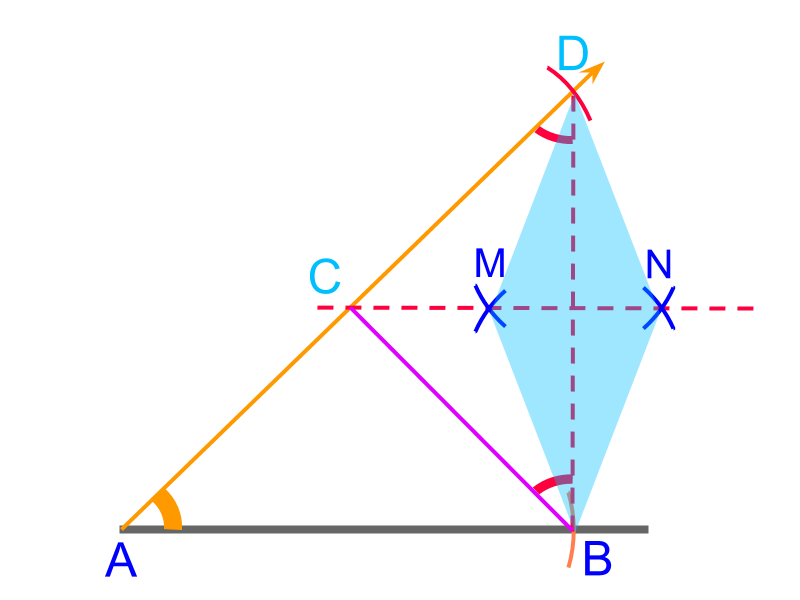Using the property that
the perpendicular bisector on the base of isosceles triangle passes through the third vertex,
The line $\stackrel{\to }{MN}$$\vec{M N}$ is drawn as bisector of $\overline{DB}$$\overline{D B}$. The point of intersection of $\stackrel{\to }{AD}$$\vec{A D}$ and $\stackrel{\to }{MN}$$\vec{M N}$ is the point $C$$C$.
$△ABC$$\triangle A B C$ is constructed.

summaryConstruction of Side-Angle-Sum of 2 Sides triangle : Visualize the point $C$$C$ and the $△BCD$$\triangle B C D$ is isosceles triangle.

Locate point $C$$C$ by one of the following methods.

•  copy the angle

•  draw perpendicular bisector on the base.

side-angle-difference between other two sides

To construct a triangle, "side-angle-difference between other two sides" is provided. The given angle with two line segments of given side and difference between sides are directly constructed using the given parameters.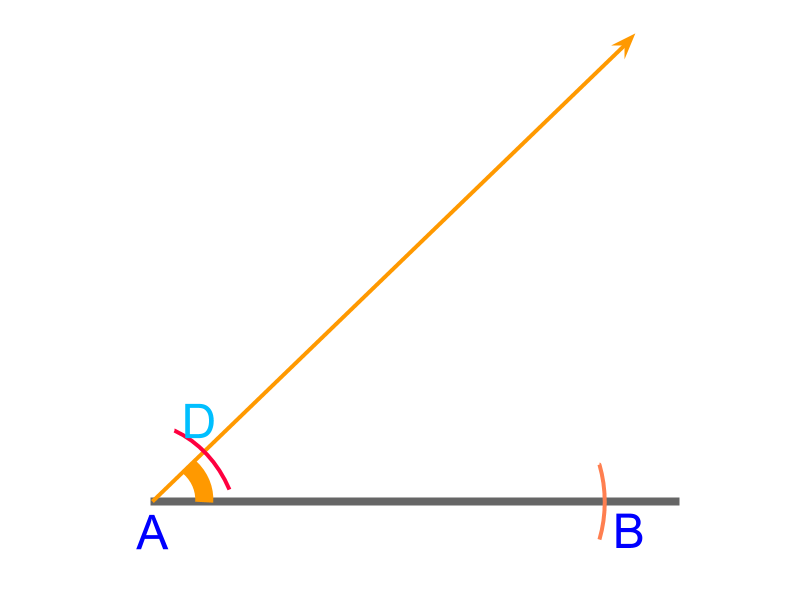To construct a triangle $△ABC$$\triangle A B C$ with given "side-angle-difference between other two sides" $\overline{AB}=4$$\overline{A B} = 4$cm, $\angle A={50}^{\circ }$$\angle A = {50}^{\circ}$, and $\overline{AC}-\overline{BC}=1$$\overline{A C} - \overline{B C} = 1$cm.

•  Construct a line and mark side $\overline{AB}$$\overline{A B}$ with a compass measuring $4$$4$cm

•  measure ${50}^{\circ }$${50}^{\circ}$ angle and draw a ray from point $A$$A$

•  measure $\overline{AC}-\overline{BC}$$\overline{A C} - \overline{B C}$ $=1$$= 1$cm in a compass and draw an arc cutting the ray at $D$$D$

This is directly constructed from the given parameters.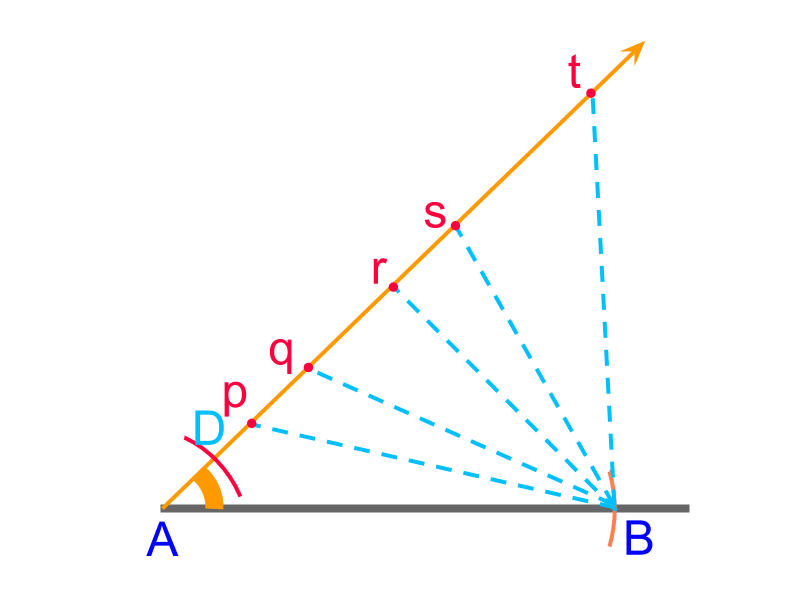The objective now is to locate the point $C$$C$ in the line $\stackrel{\to }{AD}$$\vec{A D}$.

Consider points $p$$p$, $q$$q$, $r$$r$, $s$$s$, and $t$$t$ on line $\stackrel{\to }{AD}$$\vec{A D}$. The following properties are noted for points $p$$p$ and $t$$t$ in the line $\overline{AD}$$\overline{A D}$

•  $\overline{Ap}-\overline{pB}<\overline{AD}$$\overline{A p} - \overline{p B} < \overline{A D}$

•  $\overline{At}-\overline{tB}>\overline{AD}$$\overline{A t} - \overline{t B} > \overline{A D}$

•  It is noted that at a single position in $\stackrel{\to }{AD}$$\vec{A D}$, the point $C$$C$ is located such that $\overline{AC}-\overline{CB}=\overline{AD}$$\overline{A C} - \overline{C B} = \overline{A D}$.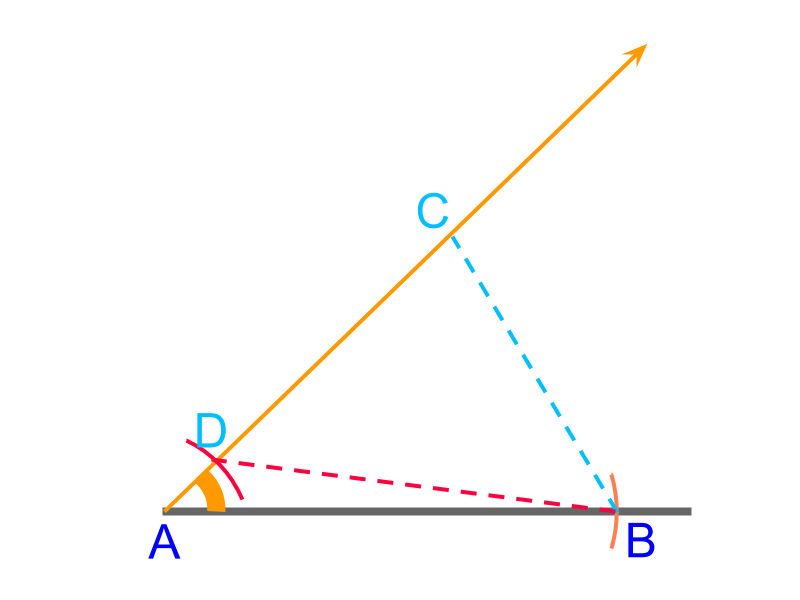The point $C$$C$ is visualized in $\stackrel{\to }{AD}$$\vec{A D}$ such that $\overline{AC}-\overline{CB}=\overline{AD}$$\overline{A C} - \overline{C B} = \overline{A D}$. The following are useful observations that will help to locate the point $C$$C$ along $\stackrel{\to }{AD}$$\vec{A D}$

•  the line segments $\overline{CD}$$\overline{C D}$ and $\overline{CB}$$\overline{C B}$ are equal

•  the triangle $△BCD$$\triangle B C D$ is isoscelesThe position of point $C$$C$ is not yet marked. It is shown in figure to visualize. The following properties are helpful in identifying the point $C$$C$.

•  two angles of an isosceles triangles are equal. $\angle CDB=\angle DBC$$\angle C D B = \angle D B C$

•  a perpendicular bisector on the base of isosceles triangle passes through the third vertex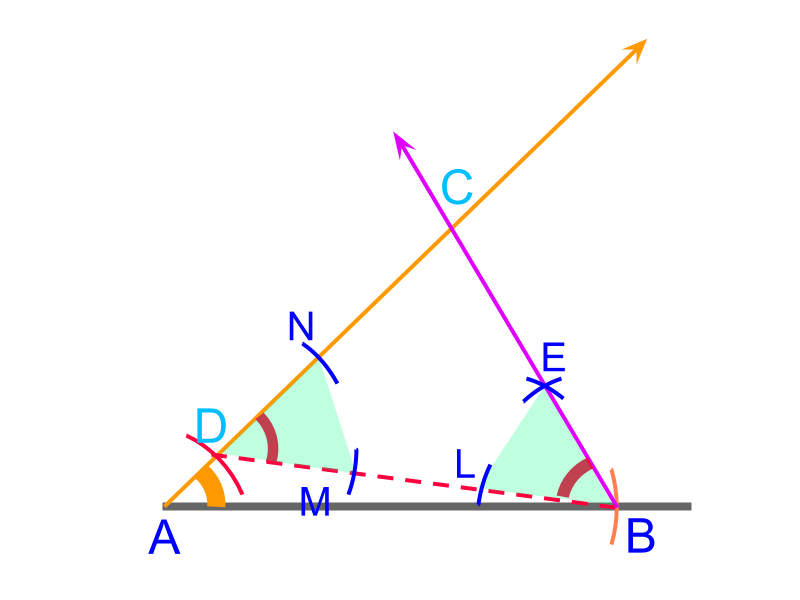Using the property that
two angles of an isosceles triangles are equal. $\angle CDB=\angle DBC$$\angle C D B = \angle D B C$
The angle $\angle CDB$$\angle C D B$ is copied using a compass to mark line $\stackrel{\to }{BE}$$\vec{B E}$

The point of intersection of rays $\stackrel{\to }{AD}$$\vec{A D}$ and $\stackrel{\to }{BE}$$\vec{B E}$ is the point $C$$C$.
$△ABC$$\triangle A B C$ is constructed.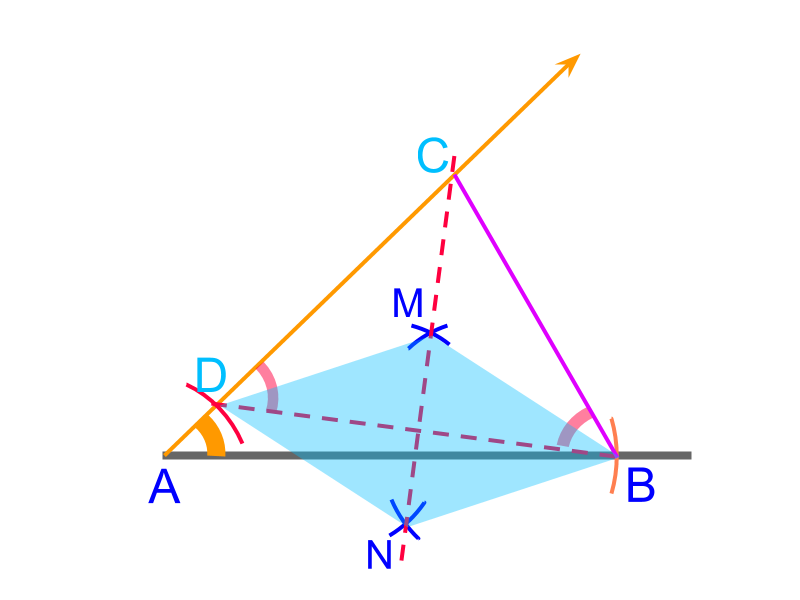Using the property that
the perpendicular bisector on the base of isosceles triangle passes through the third vertex,
The line $\stackrel{\to }{MN}$$\vec{M N}$ is constructed as the bisector of $\overline{DB}$$\overline{D B}$. The point of intersection of $\stackrel{\to }{AD}$$\vec{A D}$ and $\stackrel{\to }{MN}$$\vec{M N}$ is the point $C$$C$.
$△ABC$$\triangle A B C$ is constructed.

summaryConstruction of Side-Angle-Difference between 2 Sides of triangle :Visualize that at point $C$$C$, the $△BCD$$\triangle B C D$ is isosceles triangle.
Locate point $C$$C$ with one of the following methods.

•  copy the angle

•  draw perpendicular bisector on the base.

side-angle-difference between other two sides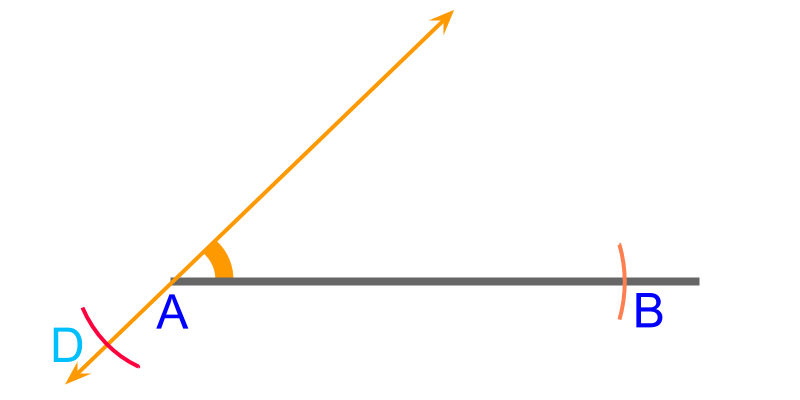To construct a triangle $△ABC$$\triangle A B C$ with given "side-angle-difference between other two sides" $\overline{AB}=4$$\overline{A B} = 4$cm, $\angle A={50}^{\circ }$$\angle A = {50}^{\circ}$, and $\overline{AC}-\overline{BC}=-1$$\overline{A C} - \overline{B C} = - 1$cm OR $\overline{BC}-\overline{AC}=1$$\overline{B C} - \overline{A C} = 1$cm.

•  Construct a line and mark $\overline{AB}$$\overline{A B}$ measuring $4$$4$cm

•  measure ${50}^{\circ }$${50}^{\circ}$ angle and draw a ray from point $A$$A$

•  measure $\overline{BC}-\overline{AC}$$\overline{B C} - \overline{A C}$ $=1$$= 1$cm and draw an arc cutting the ray at $D$$D$ at the back-end of the ray, as shown in the figure.

This is directly constructed from the given parameters.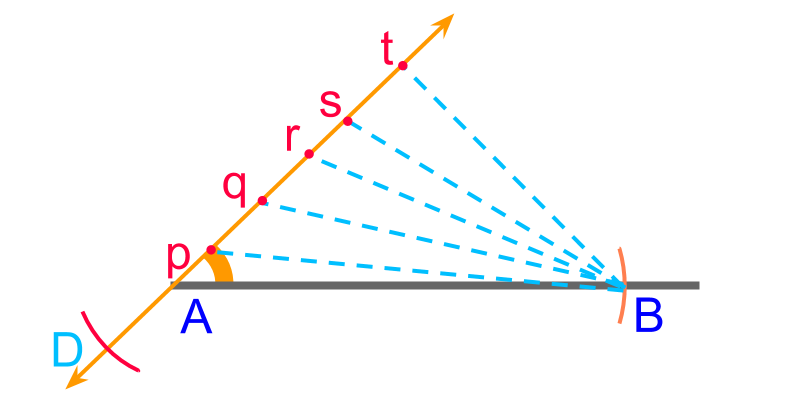The objective now is to locate the point $C$$C$ in the line $\stackrel{\to }{AD}$$\vec{A D}$.

Consider points $p$$p$, $q$$q$, $r$$r$, $s$$s$, and $t$$t$ on line $\stackrel{\to }{AD}$$\vec{A D}$. The following property is noted for points $p$$p$ and $t$$t$ in the line $\stackrel{\to }{AD}$$\vec{A D}$

•  $\overline{Ap}-\overline{pB}<\overline{AD}$$\overline{A p} - \overline{p B} < \overline{A D}$

•  $\overline{At}-\overline{tB}>\overline{AD}$$\overline{A t} - \overline{t B} > \overline{A D}$

•  It is noted that at a single position in $\stackrel{\to }{AD}$$\vec{A D}$, the point $C$$C$ is located such that $\overline{AC}-\overline{CB}=\overline{AD}$$\overline{A C} - \overline{C B} = \overline{A D}$.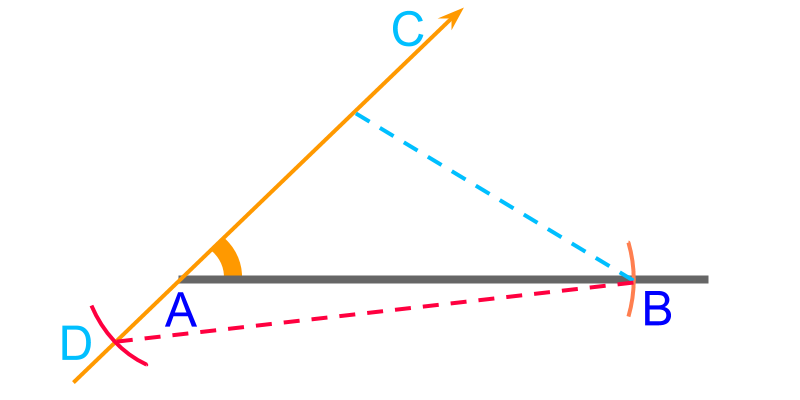The point $C$$C$ is visualized in $\stackrel{\to }{AD}$$\vec{A D}$ such that $\overline{BC}-\overline{AC}=\overline{AD}$$\overline{B C} - \overline{A C} = \overline{A D}$. The following are useful observations that will help to locate the point $C$$C$ along $\stackrel{\to }{AD}$$\vec{A D}$

•  the line segments $\overline{CD}$$\overline{C D}$ and $\overline{CB}$$\overline{C B}$ are equal

•  the triangle $△BCD$$\triangle B C D$ is isoscelesThe position of point $C$$C$ is not yet marked. It is shown in the figure to visualize. The following property helps in identifying the point $C$$C$.

•  two angles of an isosceles triangles are equal. $\angle CDB=\angle DBC$$\angle C D B = \angle D B C$

•  a perpendicular bisector on the base of isosceles triangle passes through the third vertex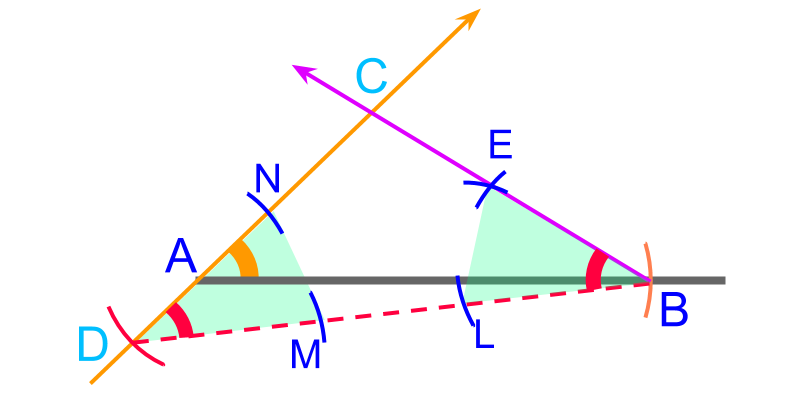Using the property that
two angles of an isosceles triangles are equal. $\angle CDB=\angle DBC$$\angle C D B = \angle D B C$
The angle $\angle CDB$$\angle C D B$ can be copied using a compass to mark line $\stackrel{\to }{BE}$$\vec{B E}$.

The point of intersection of rays $\stackrel{\to }{AD}$$\vec{A D}$ and $\stackrel{\to }{BE}$$\vec{B E}$ is the point $C$$C$.
$△ABC$$\triangle A B C$ is constructed.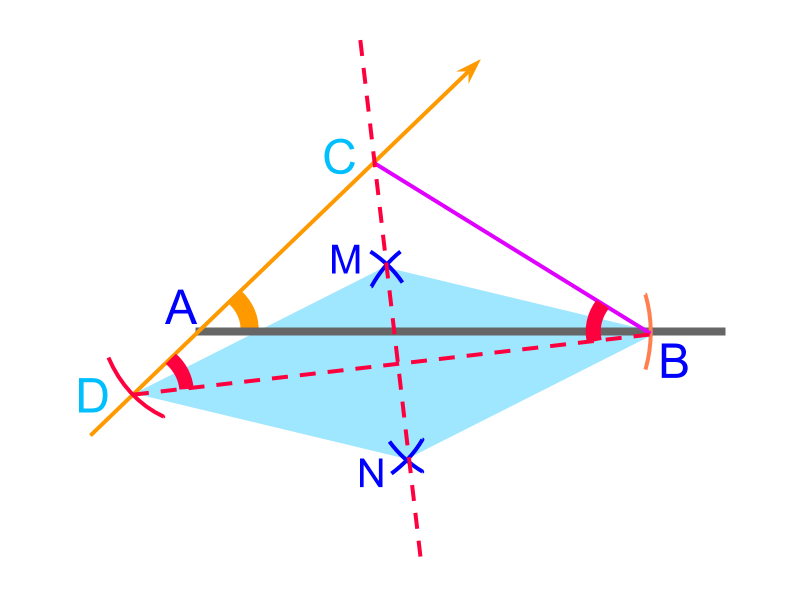Using the property that
the perpendicular bisector on the base of isosceles triangle passes through the third vertex,
The line $\stackrel{\to }{MN}$$\vec{M N}$ is constructed as the bisector of $\overline{DB}$$\overline{D B}$. The point of intersection of $\stackrel{\to }{AD}$$\vec{A D}$ and $\stackrel{\to }{MN}$$\vec{M N}$ is the point $C$$C$.
$△ABC$$\triangle A B C$ is constructed.

summaryConstruction of Side-Angle-Negative difference between 2 Sides of triangle : Visualize that at point $C$$C$, the $△BCD$$\triangle B C D$ is isosceles triangle.
Locate point $C$$C$ with one of the following methods.

•  copy the angle

•  draw perpendicular bisector on the base.

angle-angle-perimeter

To construct a triangle, "angle-angle-perimeter" is provided. Only following is directly constructed using the given parameters : a straight line, with length equivalent to the given perimeter. The given angles cannot be placed anywhere on the given straight line.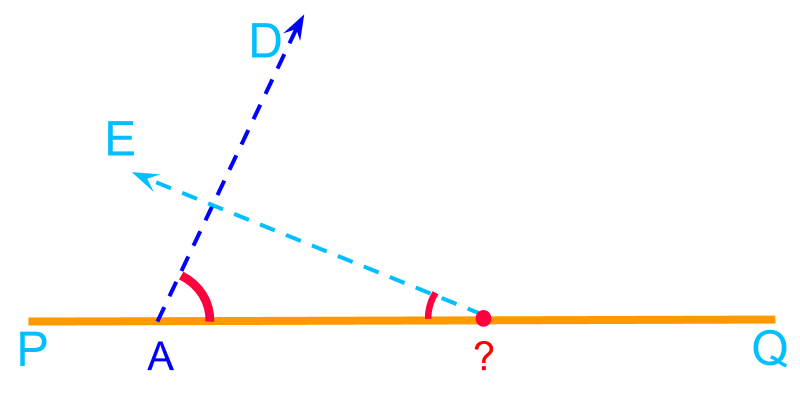To construct a triangle $△ABC$$\triangle A B C$ with given "angle-angle-perimeter" $\angle A={60}^{\circ }$$\angle A = {60}^{\circ}$, $\angle B={30}^{\circ }$$\angle B = {30}^{\circ}$, and Perimeter$=9$$= 9$cm.

•  Construct a line $\overline{PQ}$$\overline{P Q}$ measuring $9$$9$cm

This is directly constructed from the given parameters.

Point $A$$A$ is marked at an arbitrary position and locating $B$$B$ or $C$$C$ is constrained by the given two angles. The objective now is to locate the points $A$$A$ and $B$$B$ in the line $\overline{PQ}$$\overline{P Q}$ and point $C$$C$ such that the triangle has the given perimeter and the two angles.

•  Construct a line $\overline{PQ}$$\overline{P Q}$ measuring the perimeter $9$$9$cm.

•  Point $A$$A$ is visualized in an arbitrary position. It is not marked yet.

•  From the point $A$$A$, the given angle $\angle A$$\angle A$ is used to construct $\stackrel{\to }{AD}$$\vec{A D}$.

•  The position of point $B$$B$ is also visualized and the given angle $\angle B$$\angle B$ is used to construct $\stackrel{\to }{BE}$$\vec{B E}$.

The point of intersection of $\stackrel{\to }{AD}$$\vec{A D}$ and $\stackrel{\to }{BE}$$\vec{B E}$ marks point $C$$C$.

In this procedure, the positions of $A$$A$ and $B$$B$ are not known.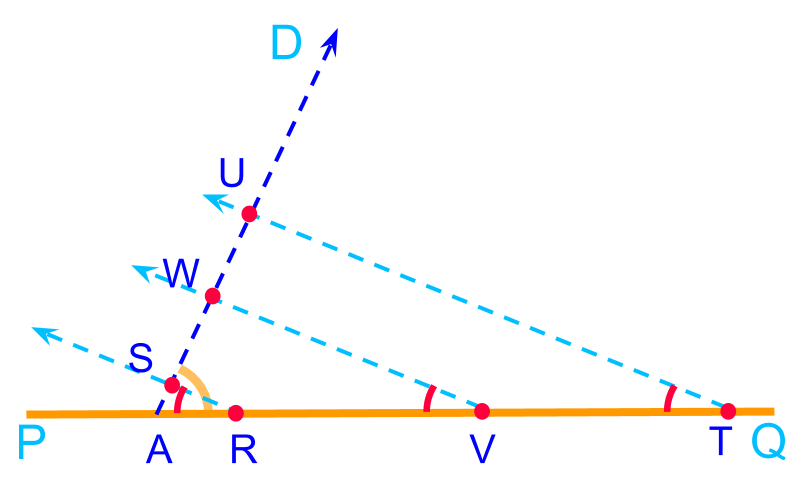Consider rays $\stackrel{\to }{rs}$$\vec{r s}$, $\stackrel{\to }{vw}$$\vec{v w}$, and $\stackrel{\to }{tu}$$\vec{t u}$. All these rays are at the given angle $\angle B$$\angle B$. These rays make triangles $△ARS$$\triangle A R S$, $△AVW$$\triangle A V W$, and $△ATU$$\triangle A T U$. These triangles have the given two angles. The following properties are noted for triangles $△ARS$$\triangle A R S$ and $△ATU$$\triangle A T U$

•  perimeter of $△ARS$$\triangle A R S$ is smaller than the given perimeter of triangle

•  perimeter of $△ATU$$\triangle A T U$ is greater than the given perimeter of triangle

•  It is noted that at only one position between the points $A$$A$ and $Q$$Q$, the point $B$$B$ is located such that perimeter of $△ABC$$\triangle A B C$ equals the given perimeter.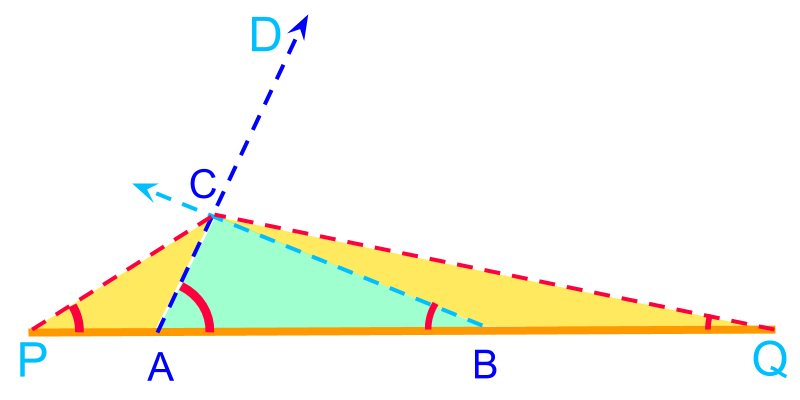The points $A$$A$, $B$$B$, and $C$$C$ are visualized such that the triangle $△ABC$$\triangle A B C$ satisfies the given perimeter and angles. The following are useful observations that will help to locate the point $C$$C$

•  the line segments $\overline{PA}$$\overline{P A}$ and $\overline{AC}$$\overline{A C}$ are equal

•  the line segments $\overline{QB}$$\overline{Q B}$ and $\overline{BC}$$\overline{B C}$ are equal

•  the triangles $△PAC$$\triangle P A C$ and $△QBC$$\triangle Q B C$ are isosceles triangles

•  the angle $\angle P$$\angle P$ is half of the given angle $\angle A$$\angle A$

•  the angle $\angle Q$$\angle Q$ is half of the given angle $\angle B$$\angle B$ These will help to locate the point $C$$C$.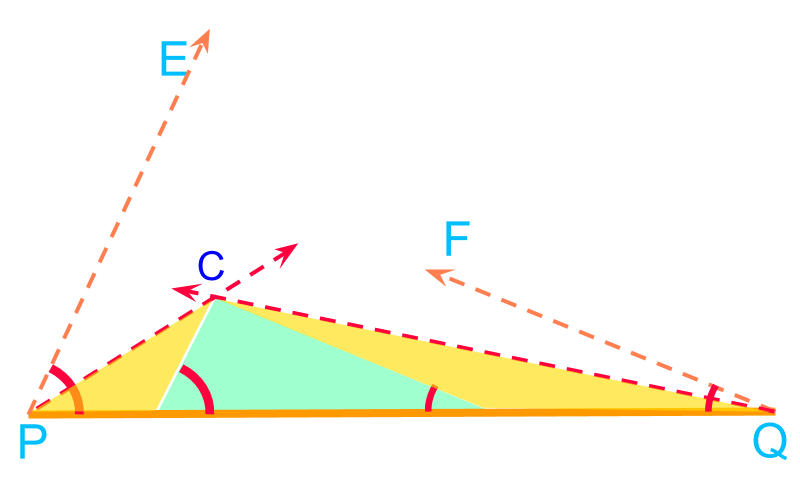It is observed that

•  $△PAC$$\triangle P A C$ and $△QBC$$\triangle Q B C$ are isosceles

•  the angle $\angle CPQ$$\angle C P Q$ is half of the given angle $\angle A$$\angle A$

•  the angle $\angle CQP$$\angle C Q P$ is half of the given angle $\angle B$$\angle B$

To construct the half angles at point $P$$P$ and point $Q$$Q$, the given angles are constructed in $\angle EPQ$$\angle E P Q$ and $\angle FQP$$\angle F Q P$ and bisected. The angle bisectors at $P$$P$ and $Q$$Q$ intersect at point $C$$C$Using the property that
$△CPA$$\triangle C P A$ and $△CQB$$\triangle C Q B$ are isosceles triangles
By copying an angle or by constructing perpendicular bisector on the base, the points $A$$A$ and $B$$B$ are marked.
$△ABC$$\triangle A B C$ is constructed.

summaryConstruction of Angle-Angle-Perimeter of triangle : Visualize that at points $A$$A$, $B$$B$, and $C$$C$, and the $△PAC$$\triangle P A C$ $△QBC$$\triangle Q B C$are isosceles triangles.

Locate point $C$$C$ by angle bisectors at point $P$$P$ and $Q$$Q$.

Locate points $A$$A$ and $B$$B$ by either copying an angle or drawing perpendicular bisector on the base.

Outline

The outline of material to learn "Consrtruction (High school)" is as follows.